International
Tables for
Crystallography
Volume B
Reciprocal space
Edited by U. Shmueli

International Tables for Crystallography (2006). Vol. B. ch. 2.5, p. 312   | 1 | 2 |

## Section 2.5.5.3. An account of absorption

B. K. Vainshteinc

#### 2.5.5.3. An account of absorption

| top | pdf |

Elastic interaction of an incident wave with a weak phase object is defined on its exit surface by the distribution of potential projection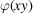; however, in the general case, the electron scattering amplitude is a complex one (Glauber & Schomaker, 1953). In such a way, the image itself has the phase and amplitude contrast. This may be taken into account if one considers not only the potential projection, but also the `imaginary potential'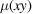which describes phenomenologically the absorption in thin specimens. Then, instead of (2.5.5.5), the wave on the exit surface of a specimen can be written as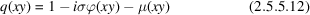and in the back focal plane if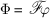and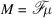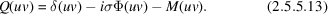Usually, μ is small, but it can, nevertheless, make a certain contribution to an image. In a sufficiently good linear approximation, it may be assumed that the real part cos χ of the phase function (2.5.5.7a)affects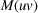, while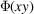, as we know, is under the action of the imaginary part sin χ.

Thus, instead of (2.5.5.6), one can write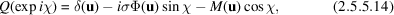and as the result, instead of (2.5.5.10),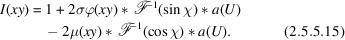The functionsandcan be separated by object imaging using the through-focus series method. In this case, using the Fourier transformation, one passes from the intensity distribution (2.5.5.15)in real space to reciprocal space. Now, at two different defocus values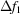and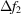[(2.5.5.6), (2.5.5.7a,b)] the values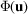and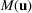can be found from the two linear equations (2.5.5.14). Using the inverse Fourier transformation, one can pass on again to real space which gives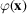and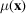(Schiske, 1968). In practice, it is possible to use several through-focus series and to solve a set of equations by the least-squares method.

Another method for processing takes into account the simultaneous presence of noise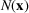and transfer function zeros (Kirkland et al., 1980). In this method the space frequencies corresponding to small values of the transfer function modulus are suppressed, while the regions where such a modulus is large are found to be reinforced.

### ReferencesGlauber, R. & Schomaker, V. (1953). The theory of electron diffraction. Phys. Rev. 89, 667–670.Google ScholarKirkland, E. J., Siegel, B. M., Uyeda, N. & Fujiyoshi, Y. (1980). Digital reconstruction of bright field phase contrast images from high resolution electron micrographs. Ultra-microscopy, 5, 479–503.Google ScholarSchiske, P. (1968). Zur Frage der Bildrekonstruktion durch Fokusreihen. 1 Y Eur. Reg. Conf. Electron Microsc. Rome, 1, 145–146.Google Scholar